# RBSE Maths Class 11 Chapter 5: Important Questions and Solutions

RBSE Maths Chapter 5 – Complex Numbers Class 11 Important questions and solutions is available here. The important questions and solutions of Chapter 5, available at BYJU’S, contain step by step explanations for the better understanding of the students. All these important questions are based on the new pattern prescribed by the RBSE. Students can also get the syllabus and textbooks on RBSE Class 11 solutions.

Chapter 5 of the RBSE Class 11 Maths will help students to solve problems related to the set of complex numbers, theorems on complex numbers, operations on complex numbers and its properties, the conjugate and properties of a complex number, modulus and properties of a complex number, geometrical representation of complex numbers and their operations, cube roots of unity, quadratic equations.

### RBSE Maths Chapter 5: Exercise 5.1 Textbook Important Questions and Solutions

Question 1: Write the following in the simplest form.

[i] i52

[ii] √-2 * √-3

[iii] (1 + i)5 * (1 – i)5

Solution:

[i] i52

abc = (ab)c

i52 = (i2)26

i2 = -1

= (-1)26

= 126

= 1

[ii] √-2 * √-3

√-2 * √-3

= √2 * √-3i

ab * ac = ab+c

i * i = i1+1

= √2 i1+1 √3

= √2i2 √3

= i2 √2 * 3

= √6i2

= -√6

[iii] (1 + i)5 * (1 – i)5

(1 + i)5 = -4 – 4i

(1 – i)5 = -4 + 4i

(-4 – 4i) * (-4 + 4i)

(a + b) (c + d) = ac + ad + bc + bd

a = -4, b = -4i, c = -4, d = 4i

= (-4) (-4) + (-4) (4i) + (-4i) (-4) + (-4i) (4i)

= 4 * 4 – 4 * 4i + 4 * 4i – 4 * 4i2

= 16 – (-16)

= 16 + 16

= 32

Question 2: Find the additive and multiplicative inverse of the following complex numbers.

[i] 1 + 2i

[ii]  / [3 + 4i]

[iii] [3 + i]2

Solution:

[i] 1 + 2i

Let z = 1 + 2i

Additive inverse = -z = – (1 + 2i) = -1 – 2i

Multiplicative inverse = [1 / z] [1 / 1 + 2i]

= [1 / 1 + 2i] * [(1 – 2i) / (1 – 2i)]

= [(1 – 2i) / (12 – (2i)2)]

= [(1 – 2i) / (1 – 4i2)]

= (1 – 2i) / (1 + 4)

= (1 – 2i) / 5

= (1 / 5) – i (2 / 5)

[ii]  / [3 + 4i]

Let z =  / [3 + 4i]

= -1 / [3 + 4i]

= [-1 / (3 + 4i)] * [(3 – 4i) / (3 – 4i)]

= [-(3 – 4i) / (32 – (4i)2)]

= [-(3 – 4i) / (9 – 16i2)]

= -(3 – 4i) / (9 + 16)

= -(3 – 4i) / 25

= -(3 / 25) + i (4 / 25)

Multiplicative inverse = [1 / z]

= (1) /  / [3 + 4i]

= 3 + 4i

[iii] [3 + i]2

Let z = [3 + i]2

= -[3 + i]2

[3 + i]2

= 32 + i2 + 6i

= 9 + i2 + 6i

= 9 – 1 + 6i

= 8 + 6i

Additive inverse = -(8 + 6i)

= -8 – 6i

Multiplicative inverse = [1 / z]

= [1 / (3 + i)2)]

= [1 / (8 + 6i)] * [(8 – 6i) / (8 – 6i)]

= [(8 – 6i) / (64 – 36i2)]

= [(8 – 6i) / (64 + 36)]

= [(8 – 6i) / 100]

= (8 / 100) – i (6 / 100)

= (2 / 25) – i (3 / 50)

Question 3: Find the conjugate of the complex number [(2 + i)3 / (3 + i)].

Solution:

Let z = (2 + i)3 / [3 + i]

Rewrite: (2 + i)3 / [3 + i] = (1 / 10) [(2 + i)3] / [(3 − i)]

i2 = −1

(2 + i)3 = 2 + 11i

[1 / 10] [(3 − i)] (2 + i)3 = [1 / 10] (3 − i) (2 + 11i)

a (b + c) = ab + ac

[1 / 10] (2 + 11i) = (1 / 10) (2) + (1 / 10) (11i)

= [1 / 5] + [11i / 10]

(3 − i) (1 / 10 (2 + 11i))

= (3 − i) (15 + 11i / 10)

((1 / 5 + 11i/ 10) (3 − i))

= (17 / 10 + 31i / 10)

Hence the conjugate of z = (17 / 10) – (31i / 10)

Question 4: Find the modulus of the following.

[i] 4 + i

[ii] -2 – 3i

[iii] 1 / [3 – 2i]

Solution:

[i] 4 + i

The modulus of a + ib is

√a2 + b2

= √42 + 12

= √16 + 1

|z| = √17

[ii] -2 – 3i

The modulus of a + ib is

√a2 + b2

= √(-2)2 + (-3)2

= √4 + 9

|z| = √13

[iii] 1 / [3 – 2i]

The modulus of a + ib is

√a2 + b2

Let z = [1 / (3 – 2i)]

= [1 / (3 – 2i)] * [(3 + 2i) / (3 + 2i)]

= [3 + 2i] / [9 – 4i2]

= [(3 + 2i) / (9 + 4)]

= [(3 + 2i) / 13]

= (3 / 13) + i (2 / 13)

Modulus of z = √a2 + b2

= √(3 / 13)2 + (2 / 13)2

= √(9 / 169) + (4 / 169)

= √13 / 169

|z| = 1 / √13

Question 5: If a2 + b2 = 1, then find the value of [1 + b + ia] / [1 + b – ia].

Solution:

[1 + b + ia] / [1 + b – ia]

= [1 + b + ia] / [1 + b – ia] * [1 + b + ia] / [1 + b + ia]

= [(1 – b)2 – a2 + 2ia (1 + b)] / [1 + b2 + 2b + a2]

= [(1 – a2) + 2b + b2 + 2ia (1 + b)] / [2(1 + b)]

= [2b2 + 2b + 2ia (1 + b)] / [2 (1 + b)]

= b + ia

Question 6: If a = cos θ + i sin θ, then find the value of [1 + a] / [1 – a].

Solution:

A = cosθ + isinθ

[1 + a] / [1 – a]

= (1 + cosθ + isinθ) / (1 – cosθ – isinθ)

= (1 + cosθ + isinθ) (1 – cosθ + isinθ) / (1 – cosθ + isinθ) (1 – cosθ – isinθ)

= (1 + cosθ + isinθ – cosθ – cos²θ – isinθ cosθ + isinθ + isinθ cosθ + i² sin²θ) / {(1 – cosθ)² – (isinθ)²}

= (1 + 2 isinθ – cos²θ – sin²θ) / {1 – 2 cosθ + cos²θ – (i² sin²θ)}

= {1 + 2 isinθ – (sin²θ + cos²θ)} / (1 – 2 cosθ + cos²θ + sin²θ)

= (1 + 2isinθ – 1) / (1 – 2cosθ + 1)

= 2 isinθ / (2 – 2 cosθ)

= isinθ / (1 – cosθ)

= i (2 sinθ / 2 cos θ/2) / (2 sin² θ/2)

= (i cos θ/2) / (sin θ/2)

= i cot θ/2

Question 7: Find the value of x and y which satisfy the equation {[(1 + i) x – 2i] / [3 + i]} + {[(2 – 3i) y + i] / [3 – i]} = i.

Solution:

{[(1 + i) x – 2i] / [3 + i]} + {[(2 – 3i) y + i] / [3 – i]}

{[(1 + i) x – 2i] (3 – i) + [(2 – 3i) y + i]} / 32 – i2 = i

[x + ix – 2i] (3 – i) + [2y – 3iy + i] (3 + i) = 10i

3x + 3ix – 6i – ix – i2x + 2i2 + 6y – 9iy + 3i + 2iy – 3i2y + i2 = 10i

3x + 3ix – 6i – ix + x – 2 + 6y – 9iy + 3i + 2iy + 3y – 1 = 10i

3x + 3ix – 6i – ix + x – 2 + 6y – 9iy + 3i + 2iy + 3y – 1 – 10i = 0

(4x + 9y – 3) + i (3x – 6 – x – 9y + 3 + 2y – 10) = 0

(4x + 9y – 3) + i (2x – 7y – 13) = 0

(4x + 9y – 3) —- (1)

(2x – 7y – 13) —- (2)

Equate the real and imaginary parts,

Multiply the second equation by 2 and subtract it from the first equation.

4x + 9y – 4x + 14y = 3 – 26

23y = -23

y = -1

Substitute y = -1 in (1), we get x = 3

Hence x = 3 and y = -1.

Question 8: If z1 and z2 are any two complex numbers, then prove that

|z1 + z2|2 + |z1 – z2|2 = 2 |z1|2 + 2 |z2|2.

Solution:

LHS = |z1 + z2|2 + |z1 – z2|2

= |z1|2 + |z2|2 + 2 Re (z1 z2) + |z1|2 + |z2|2 – 2 Re (z1 z2)

= 2 |z1|2 + 2 |z2|2 + 2 Re (z1 z2) – 2 Re (z1 z2)

= 2 |z1|2 + 2 |z2|2

= RHS

|z1 + z2|2 + |z1 – z2|2 = 2 |z1|2 + 2 |z2|2

Question 9: If a + ib = [c + i] / [c – i], where c is a real number, then prove that a2 + b2 = 1 and [b / a] = [2c] / [c2 – 1].

Solution:

a + ib = [c + i] / [c – i]

= (c + i) (c + i) / (c – i) (c + i)

= (c² + 2ci + i²) / (c² – i²)

= (c² + 2ci – 1) / {c² – (-1)}

= {(c² – 1) + 2ci} / (c² + 1)

a + ib = (c² – 1) / (c² + 1) + i (2c) / (c² + 1)

Equating the real and imaginary part from both sides we get,

a = c² – 1 / c ² + 1 and b = 2c / c² + 1

a² + b²

= {(c² – 1) / (c² + 1)}² + {2c / (c² + 1)}²

= {(c² – 1)² + 4c²} / (c² + 1)²

= (c⁴ – 2c² + 1 + 4c²) / (c² + 1)²

= (c⁴ + 2c² + 1) / (c² + 1)²

= (c² + 1)² / (c² + 1)²

= 1

Now, b / a

= {2c / (c² + 1)} / {(c² – 1) / (c² + 1)}

= 2c / (c² + 1) * (c² + 1) / (c² – 1)

= 2c / (c² – 1)

Question 10: If (x + iy) = a + ib, then prove that [x / a] + [y / b] = 4 (a2 – b2).

Solution:

(x + iy) = a + ib

→ x + iy = (a + ib)³

→ x + iy = a³ + 3a²bi – 3ab² – ib³

→ (x + iy) = (a³ – 3ab²) + (3a² – b³) i

By the equality of complex numbers:

x = a (a² – 3b²); y = b (3a² – b²)

[x / a] = a2 − 3b2 ….. (1)

[y / b] = 3a2 − b2 ….. (2)

Adding (1) and (2) we get,

[x / a] + [y / b] = 4 (a2 – b2)

Question 11: If [x + i]2 / [3x + 2] = a + ib, then prove that [x2 + 1]2 / [3x + 2]2 = a2 + b2.

Solution: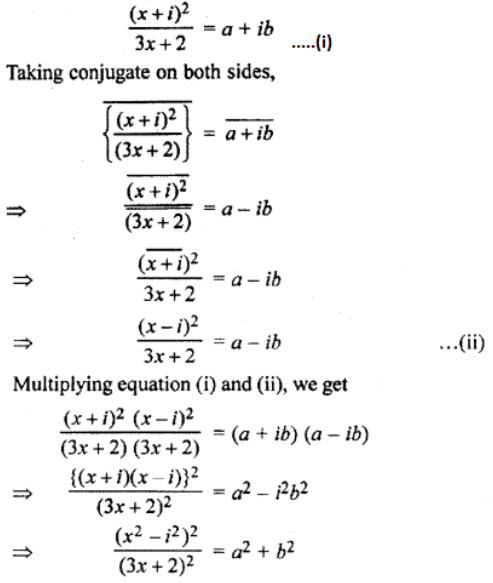### RBSE Maths Chapter 5: Exercise 5.2 Textbook Important Questions and Solutions

Question 1: Find the arguments of the following numbers.

[i] [1 + i] / [1 – i]

[ii] -1 + √3i

[iii] [5 + i √3] / [4 – i 2√3]

Solution:

[i] z = [1 + i] / [1 – i] * [1 + i] / [1 + i]

= [1 + i2 + 2i] / [1 + 1]

= i

= 0 + i

In polar form,

r (cosθ + i sinθ) = 0 + i

⇒ r cosθ = 0 and r sinθ = 1

⇒ r2 (cos2θ + sin2θ) = 02 + 12

⇒ r2 = 1

⇒ r = 1

Now, cosθ = 1

⇒ θ = π / 2

The argument is π / 2 and modulus is 1.

[ii] -1 + √3i

a = -1, b = √3

z = -1 + √3i = (-1, √3) lies in the second quadrant.

Argument θ = π – tan-1 |(b / a)|

= π – tan-1 |(√3 / -1)|

= π – tan-1 (√3)

= π – [π / 3]

= 2π / 3

[iii] [5 + i √3] / [4 – i 2√3]

1 / [a + ib] = {1 / [(a – ib) (a + ib)]} * (a – ib)

= [a – ib] / a2 + b2

On splitting,

[a – ib] / a2 + b2 = [a / a2 + b2] – [ib / a2 + b2]

Here a = 4, b = -2√3

[5 + i √3] *  / [4 – i 2√3] = [5 + i √3] ([1 / 7] + [i √3 / 2]

([1 / 7] + [i √3 / 2] * [5 + i √3] = ([1 / 2] + [i √3 / 2]

For a complex number a + ib, the polar form is given by r [cos θ + i sin θ] where, r = √a2 + b2 and θ = a tan [b / a]

a = [1 / 2] and b = √3 / 2

= [1 / 2] tan ([√3 / 2] / [1 / 2])

= π / 3

Question 2: Express the following complex numbers in polar form.

[i] [1 + i] / √2

[ii] sin [π / 3] + i cos [π / 3]

Solution:

[i] [1 + i] / √2

For a complex number a + ib, the polar form is given by r [cos θ + i sin θ] where, r = √a2 + b2 and

a = [√2 / 2] and b = √2 / 2

r = √[√2 / 2]2 + [√2 / 2]2

= 1

θ = a tan [b / a]

= [√2 / 2] tan ([√2 / 2] / [√2 / 2])

= π / 4

[√2 / 2] + i [√2 / 2] = cos[π / 4] + i sin [π / 4] [ii] sin [π / 3] + i cos [π / 3]

For a complex number a + ib, the polar form is given by r [cos θ + i sin θ] where, r = √a2 + b2 and

a = [√3 / 2] and b = 1 / 2

r = √[√3 / 2]2 + [1 / 2]2

= 1

θ = a tan [b / a]

= [√3 / 2] tan ([1 / 2] / [√3 / 2])

= π / 6

[√3 / 2] + i [1 / 2] = cos[π / 6] + i sin [π / 6]

Question 3: If z1 and z2 are two non-zero complex numbers, then prove that arg z1 = arg z1 – arg z2.

Solution: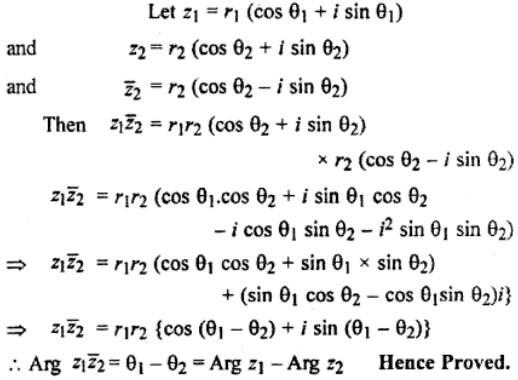### RBSE Maths Chapter 5: Exercise 5.3 Textbook Important Questions and Solutions

Question 1: Find the square root of the following complex numbers.

[i] -5 + 12i

[ii] 8 – 6i

[iii] -i

Solution:

[i] -5 + 12i

If a + ib = -5 + 12i

Here a = -5, b = 12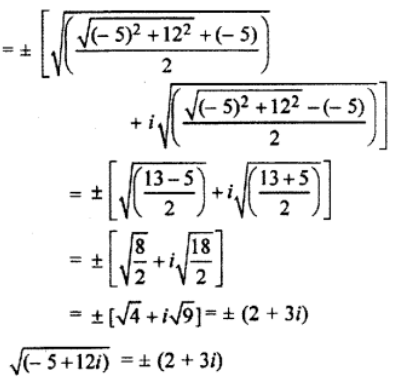[ii] 8 – 6i

If a + ib = 8 + 6i

Here a = 8, b = 6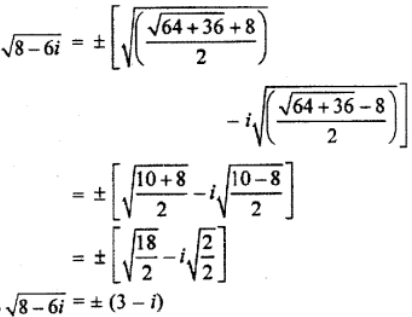[iii] -i

If a – ib = 0 – i

Here a = 0, b = 1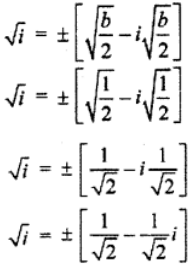Question 2: Find the value of √[4 + 3 √-20] + √[4 – 3 √-20].

Solution: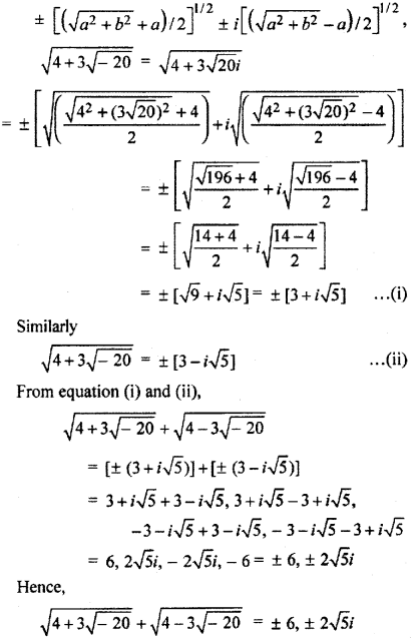Question 3: Find the cube root of the following.

[i] -216

[ii] -512

Solution:

(i) -216 = (-6) (1)1/3

= -6, -6ω, -6ω2

Hence, cube root of -216 = -6, -6ω, -6ω2

(ii) -512 = (-8) (1)1/3

= -8, -8ω, -8ω2

Hence, cube root of -512 = -8, -8ω, – 8ω2

Question 4: Prove that:

(i) 1 + ωn + ω2n = 0, whereas n = 2, 4.

(ii) 1 + ωn + ω2n = 3, whereas n is multiple of 3.

Solution:

[i] 1 + ωn + ω2n = 0, whereas n = 2, 4.

When n = 2,

1 + ωn + ω2n = 1 + ω2 + ω2*2

= 1 + ω2 + ω4

= 1 + ω2 + ω * ω3

= 1 + ω2 + ω

= 1 + ω + ω2

= 0

When n = 4,

1 + ωn + ω2n = 1 + ω4 + ω2*4

= 1 + ω * ω3 + ω3 * ω3 * ω2

= 1 + ω * 1 + 1 * 1 * ω2

= 1 + ω + ω2

= 0

[ii] 1 + ωn + ω2n = 3, whereas n is multiple of 3.

n = 3k

1 + ωn + ω2n = 1 + ω3k + ω2 * 3k

= 1 + (ω3)k + (ω3)2k

= 1 + (1)k + (1)2k

= 1 + 1 + 1

= 3

Question 5: Prove that:

[i] [(-1 + √-3) / 2]29 + [(-1 – √-3) / 2]29 = -1

[ii] (1 + 5ω2 + ω) (1 + 5ω + ω2 ) (5 + ω + ω2 ) = 64

Solution:

[i] [(-1 + √-3) / 2]29 + [(-1 – √-3) / 2]29 = -1

ω = [(-1 + i√3) / 2] and ω2 = [(-1 – i√3) / 2]

LHS = [(-1 + √-3) / 2]29 + [(-1 – √-3) / 2]29

= [(-1 + i√3) / 2]29 + [(-1 – i√3) / 2]29

= (ω)29 + (ω2)29

= (ω3)9 ω2 + ω58

= ω2 + (1)19 ω

= ω2 + ω

= -1

[ii] (1 + 5ω2 + ω) (1 + 5ω + ω2 ) (5 + ω + ω2) = 64

LHS = (1 + 5ω2 + ω) (1 + 5ω + ω2 ) (5 + ω + ω2)

= (1 + ω + ω2 + 4ω2) (1 + ω + ω2 + 4ω) (1 + ω + ω2 + 4)

= (0 + 4ω2) (0 + 4ω) (0 + 4)

= 4ω2 * 4ω * 4

= 64ω3

= 64 * 1

=64

### RBSE Maths Chapter 5: Exercise 5.4 Textbook Important Questions and Solutions

Question 1: Find the solution of the following equations by vedic method.

(i) x2 + 4x + 13 = 0

(ii) 2x2 + 5x + 4 = 0

(iii) ix2 + 4x – 15 / 2 = 0

Solution:

(i) x2 + 4x + 13 = 0

Comparing this equation with ax2 + bx + c = 0, we get

a = 1, b = 4, c = 13

First derivative = Discriminant

D1 = D

2x + 4 = ± √b2 – 4ac

2x + 4 = ± √42 – 4 * (1) * (13)

2x + 4 = ± √16 – 52

2x + 4 = ± √-36

2x = -4 ± 6i

x = -2 ± 3i

The solutions of x2 + 4x + 13 = 0 are -2 ± 3i.

(ii) 2x2 + 5x + 4 = 0

Comparing this equation with ax2 + bx + c = 0, we get

a = 2, b = 5, c = 4

First derivative = Discriminant

D1 = D

4x + 5 = ± √b2 – 4ac

4x + 5 = ± √52 – 4 * (2) * (4)

4x + 5 = ± √25 – 32

4x = -5 ± √7i

x = [-5 ± √7i] / 4

(iii) ix2 + 4x – 15 / 2 = 0

2ix2 + 8x – 15 = 0

Comparing this equation with ax2 + bx + c = 0, we get

a = 2i, b = 8, c = (-15)

First derivative = Discriminant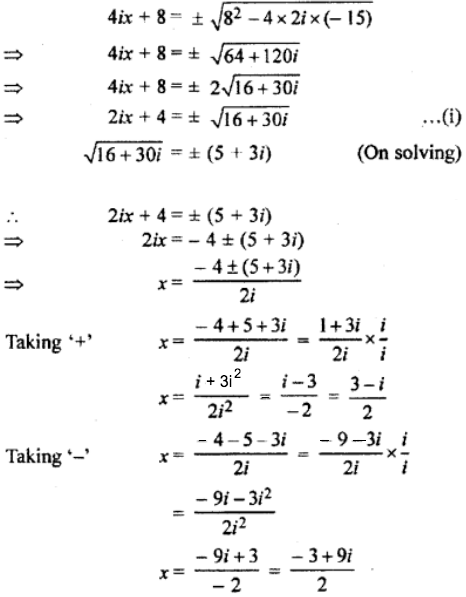Question 2: Find the quadratic equations which have the following roots:

(i) 5 and -2

(ii) 1 + 2i

Solution:

(i) Root α = 5 and β = – 2

Then, sum of roots

= α + β

= 5 – 2

= 3 and

Product of roots

= αβ

= 5 × (-2)

= -10

Hence, the required equation whose roots are 5 and -2 is

x2 – (sum of roots) x + product of roots = 0

⇒ x2 – 3x + (-10) = 0

⇒ x2 – 3x – 10 = 0

(ii) Roots α = 1 + 2i and β = 1 – 2i

Then, sum of roots

= α + β

= 1 + 2i + 1 – 2i

= 2 and

Product of roots

= αβ

= (1 + 2i) * (1 – 2i)

= 1 – 4i2

= 1 + 4

= 5

Hence, the required equation whose roots are 1 + 2i and 1 – 2i is

x2 – (sum of roots) x + product of roots = 0

⇒ x2 – 2x + 5 = 0.

Question 3: If one root of equation x2 – px + q = 0 is twice the other then prove that 2p2 = 9q.

Solution:

The given equation is x2 – px + q = 0.

If its roots are α and β, then according to question α = 2β then

Sum of roots = α + β

α + β = [-b / a]

= – (-p / 1)

2β + β = p

3β = p —— (1)

Product of roots = αβ

αβ = [c / a]

2β * β = q

2 = q

LHS = 2p2

= 2 * (3β)2

= 2 * 9β2

= 18β2

= 9 * 2β2

= 9q

= RHS

Question 4: Find that condition for which equation ax2 + bx + c = 0 has roots in the ratio m : n.

Solution:

The given equation is ax2 + bx + c = 0.

If its roots are α and β, then according to question,

α : β = m : n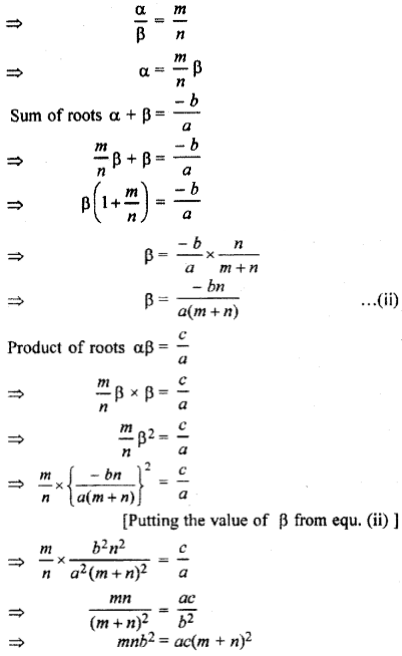Question 5: If 2 + (2a + 5ib) = 8 + 10i, then find the values of a and b

Solution:

2 + (2a + 5ib) = 8 + 10i

⇒ (2 + 2a) + 5bi = 8 + 10i

On comparing,

2 + 2a = 8

⇒ 2a = 6

⇒ a = 3 and

5b = 10

⇒ b = 2

So, a = 3 and b = 2.